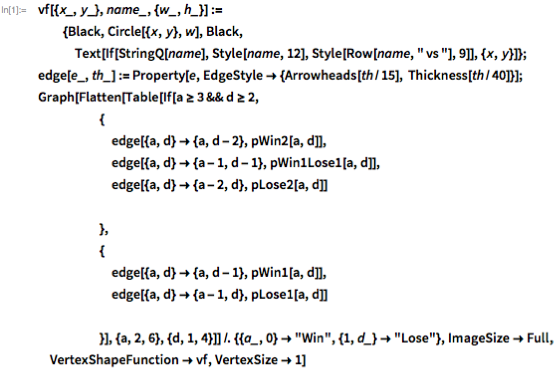Browse by Topic

# How to Win at Risk: Exact Probabilities

The classic board game Risk involves conquering the world by winning battles that are played out using dice. There are lots of places on the web where you can find out the odds of winning a battle given the number of armies that each player has. However, all the ones that I have seen do this by Monte Carlo simulation, and so are innately approximate. The Wolfram Language makes it so easy to work out the exact values that I couldn’t resist calculating them once and for all.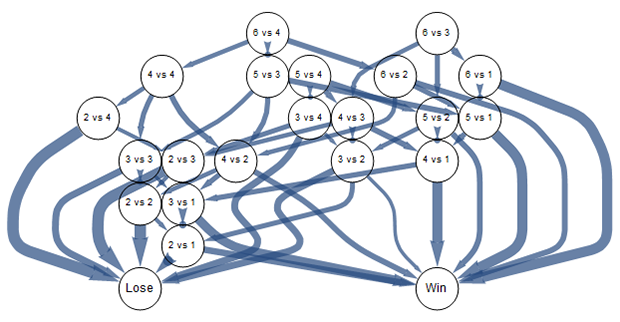Here are the basic battle rules: the attacker can choose up to three dice (but must have at least one more army than dice), and the defender can choose up to two (but must have at least two armies to use two). To have the best chances of winning, you always use the most dice possible, so I will ignore the other cases. Both players throw simultaneously and then the highest die from each side is paired, and (if both threw at least two dice) the next highest are paired. The highest die kills an army and, in the event of a draw, the attacker is the loser. This process is repeated until one side runs out of armies.

So my goal is to create a function pBattle[a,d] that returns the probability that the battle ends ultimately as a win for the attacker, given that the attacker started with a armies and the defender started with d armies.

I start by coding the basic game rules. The main case is when both sides have enough armies to fight with at least two dice. There are three possible outcomes for a single round of the battle. The attacker wins twice or loses twice, or both sides lose one army. The probability of winning the battle is therefore the sum of the probabilities of winning after the killed armies are removed multiplied by the probability of that outcome.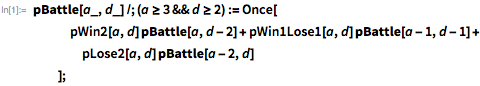We also have to cover the case that either side has run low on armies and there is only one game piece at stake.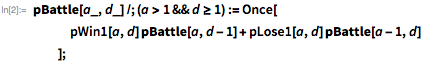This sets up a recursive definition that defines all our battle probabilities in terms of the probabilities of subsequent stages of the battle. Once prevents us working those values out repeatedly. We just need to terminate this recursion with the end-of-battle rules. If the attacker has only one army, he has lost (since he must have more armies than dice), so our win probability is zero. If our opponent has run out of armies, then the attacker has won.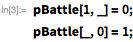Now we have to work out the probabilities of our five individual attack outcomes: pWin2, pWin1Lose1, pLose2, pWin1 and pLose1.

When using two or three dice, we can describe the distribution as an OrderDistribution of a DiscreteUniformDistribution because we always want to pair the highest throws together.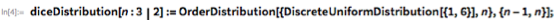For example, here is one outcome of that distribution; the second number will always be the largest, due to the OrderDistribution part.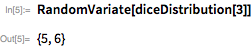The one-die case is just a uniform distribution; our player has to use the value whether it is good or not. However, for programming convenience, I am going to describe a distribution of two numbers, but we will never look at the first.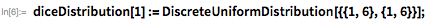So now the probability of winning twice is that both attacker dice are greater than both defenders. The defender must be using two dice, but the attacker could be using two or three.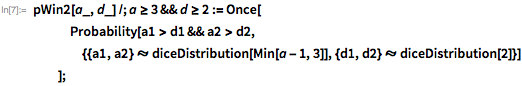The lose-twice probability has a similar definition.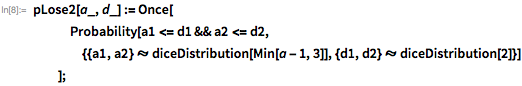And the draw probability is what’s left.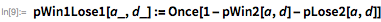The one-army battle could be because the attacker is low on armies or because the defender is. Either way, we look only at the last value of our distributions.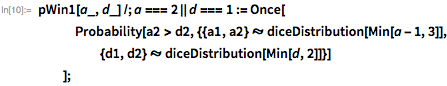And pLose1 is just the remaining case.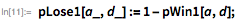And we are done. All that is left is to use the function. Here is the exact (assuming fair dice, and no cheating!) probability of winning if the attacker starts with 18 armies and the defender has only six.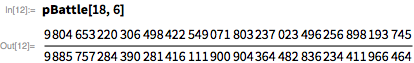We can approximate this to 100 decimal places.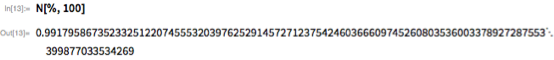We can quickly enumerate the probabilities for lots of different starting positions.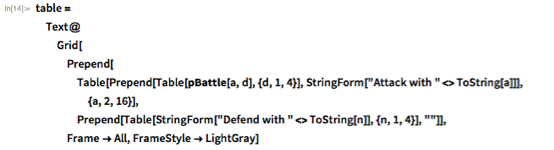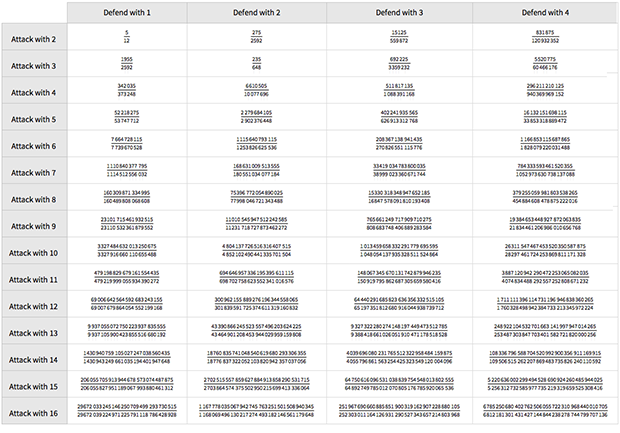Here are the corresponding numeric values to only 20 decimal places.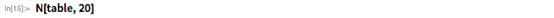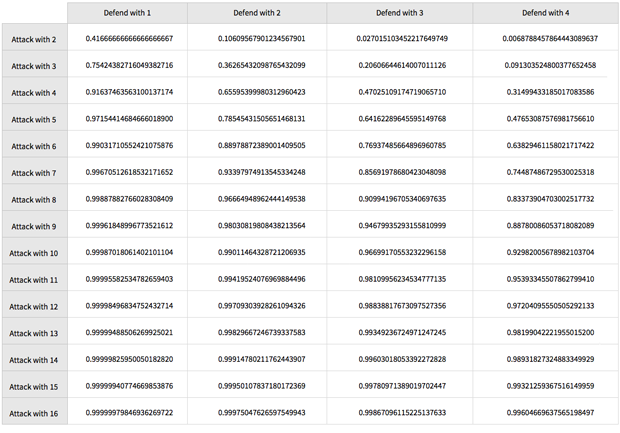You can download tables of more permutations here, with exact numbers, and here, approximated to 20 digits.

Of course, this level of accuracy is rather pointless. If you look at the 23 vs. 1 battle, the probability of losing is about half the probability that you will actually die during the first throw of the dice, and certainly far less than the chances of your opponent throwing the board in the air and refusing to play ever again.

## Appendix: Code for Generating the Outcomes Graph